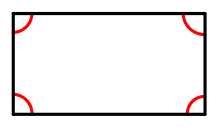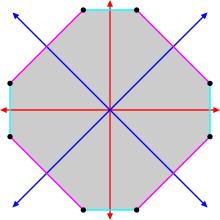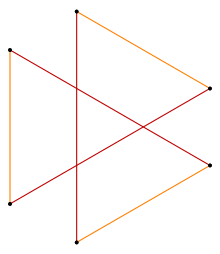# Equiangular polygonA rectangle is an equiangular quadrilateralA convex equiangular octagon with 4-fold reflective symmetryA nonconvex equiangular hexagon with 3-fold reflectional symmetry

In Euclidean geometry, an equiangular polygon is a polygon whose vertex angles are equal. If the lengths of the sides are also equal (that is, if it is also equilateral) then it is a regular polygon. Isogonal polygons are equiangular polygons which alternate two edge lengths.

## Properties

The only equiangular triangle is the equilateral triangle. Rectangles, including the square, are the only equiangular quadrilaterals (four-sided figures).

For a convex equiangular n-gon each internal angle is 180(1-2/n)°; this is the equiangular polygon theorem.

Viviani's theorem holds for equiangular polygons:

The sum of distances from an interior point to the sides of an equiangular polygon does not depend on the location of the point, and is that polygon's invariant.

A rectangle (equiangular quadrilateral) with integer side lengths may be tiled by unit squares, and an equiangular hexagon with integer side lengths may be tiled by unit equilateral triangles. Some but not all equilateral dodecagons may be tiled by a combination of unit squares and equilateral triangles; the rest may be tiled by these two shapes together with rhombi with 30 and 150 degree angles.

A cyclic polygon is equiangular if and only if the alternate sides are equal (that is, sides 1, 3, 5, ... are equal and sides 2, 4, ... are equal). Thus if n is odd, a cyclic polygon is equiangular if and only if it is regular.

For prime p, every integer-sided equiangular p-gon is regular. Moreover, every integer-sided equiangular pk-gon has p-fold rotational symmetry.

An ordered set of side lengthsgives rise to an equiangular n-gon if and only if either of two equivalent conditions holds for the polynomialit equals zero at the complex valueit is divisible by## References

1. Ball, Derek (2002), "Equiangular polygons", The Mathematical Gazette, 86 (507): 396–407, JSTOR 3621131.
2. De Villiers, Michael, "Equiangular cyclic and equilateral circumscribed polygons", Mathematical Gazette 95, March 2011, 102-107.
3. McLean, K. Robin. "A powerful algebraic tool for equiangular polygons", Mathematical Gazette 88, November 2004, 513-514.
4. M. Bras-Amorós, M. Pujol: "Side Lengths of Equiangular Polygons (as seen by a coding theorist)", The American Mathematical Monthly, vol. 122, n. 5, pp. 476–478, May 2015. ISSN 0002-9890.
• Williams, R. The Geometrical Foundation of Natural Structure: A Source Book of Design. New York: Dover Publications, 1979. p. 32